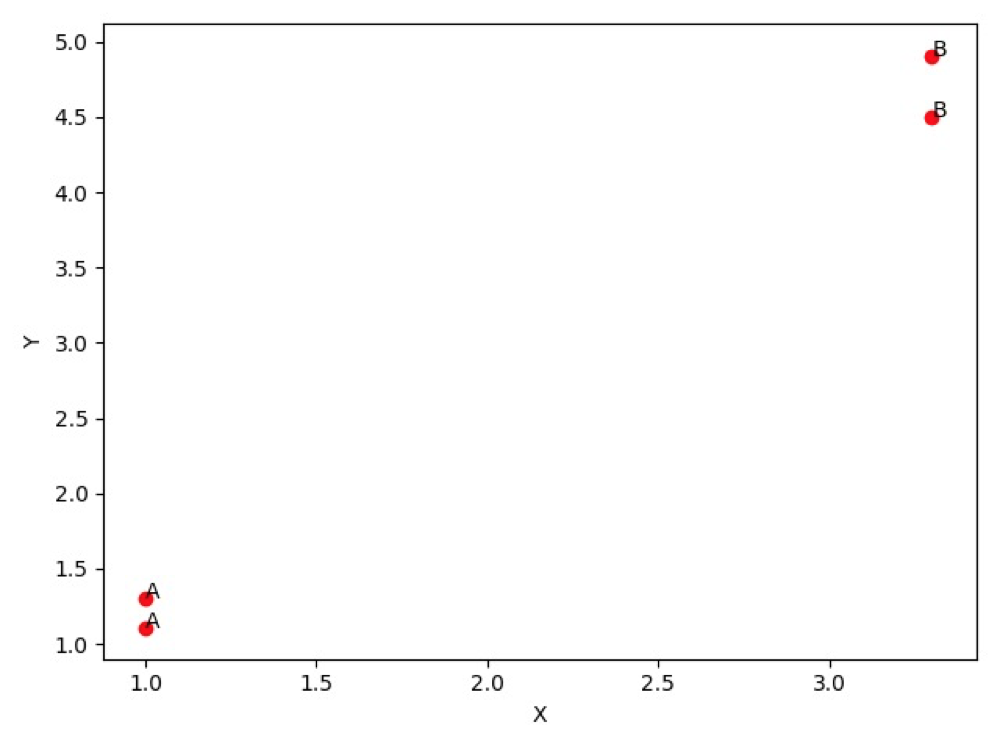# 浅读《机器学习实战》

##### Jan 09, 2020 - MachineLearn

• 监督学习

• k-近邻算法（`KNN`
• 朴素贝叶斯算法
• 支持向量机
• 决策树
• 线性回归
• 局部加权线性回归
• Ridge 回归
• Lasso 最小回归系数估计

• 非监督学习

• K-均值
• DBSCAN
• 最大期望算法
• Parzen 窗设计

## KNN

kNN（k-Nearest Neighbor）算法的核心思想是如果一个样本在特征空间中的 k 个最相邻的样本中的大多数属于某一个类别，则该样本也属于这个类别

`KNN` 是机器学习中最简单易懂的算法，它的适用面很广，并且在样本量足够大的情况下准确度很高，多年来得到了很多的关注和研究，K-近邻算法采用测量不同特征值之间的距离方法进行分类。适用数据范围：数值型和标称型

`kNN` 当中的 k 取不同值时，分类结果可能会有显著不同。

• 优点：简单，易于理解，易于实现，精度高、对异常值不敏感、无数据输入假定。
• 缺点：计算复杂度高、空间复杂度高。

``````import numpy as np
import matplotlib.pyplot as plt

# 4 个测试数据点和已经分好类的数组
group = np.array([[1.0, 1.1], [1.0, 1.3], [3.3, 4.5], [3.3, 4.9]])
labels = ['A', 'A', 'B', 'B']

def data_set_plt():

for i in range(len(labels)):
x = group[i]
y = group[i]
ax.scatter(x, y, c='r')
ax.text(x, y, labels[i])

plt.xlabel('X')
plt.ylabel('Y')
plt.show()

if __name__ == '__main__':

data_set_plt()

``````### 欧氏距离公式

`KNN` 需要计算样本之间的距离，需要用到欧氏距离公式``````def classify(input, dataSet, labels, k):
dataSize = dataSet.shape

# 计算欧式距离
diff = np.tile(input, (dataSize, 1)) - dataSet
sqdiff = diff ** 2
squareDist = np.sum(sqdiff, axis=1)  # 行向量分别相加，从而得到新的一个行向量
dist = squareDist ** 0.5

# 对距离进行排序
sortedDistIndex = np.argsort(dist)  # argsort()根据元素的值从大到小对元素进行排序，返回下标

classCount = {}
for i in range(k):
voteLabel = labels[sortedDistIndex[i]]
# 对选取的K个样本所属的类别个数进行统计
classCount[voteLabel] = classCount.get(voteLabel, 0) + 1
# 选取出现的类别次数最多的类别
maxCount = 0
for key, value in classCount.items():
if value > maxCount:
maxCount = value
classes = key

return classes

``````

``````classify([0,0], group, labels, 3)
``````

TBD